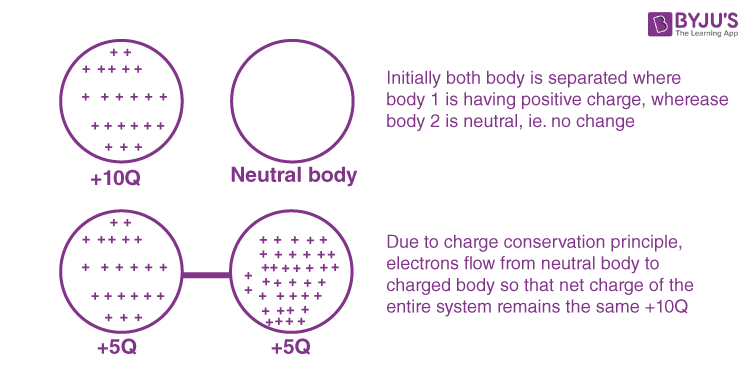# Conservation of Charge

## What is Conservation of Charge?

A charge is a property associated with the matter due to which it produces and experiences electrical and magnetic effects. The basic idea behind the conservation of charge is that the total charge of the system is conserved. We can define it as:

Conservation of Charge is the principle that the total electric charge in an isolated system never changes. The net quantity of electric charge, the amount of positive charge minus the amount of negative charge in the universe, is always conserved.

As we know, the system is the group of objects and its interaction with charges is similar to the conservation of energy and momentum, But this conservation law is more intuitive because the net charge of an object depends on the number of electrons and protons. The protons and electron cannot just appear or disappear out of nowhere, the total charge has to be the same. That’s the reason there is always the same number of electrons and protons in a body.

It is known that every atom is electrically neutral, containing as many electrons as the number of protons in the nucleus. Bodies can also have any whole multiples of the elementary charge:

Electrical charge resides in electrons and protons, the smallest charge that a body can have is the charge of one electron or proton. [ie. – 1.6 x 10-19 C  or + 1.6 x 10-19 C]

### Explanation:

The law of conservation of charge says that the net charge of an isolated system will always remain constant.  Let’s try to understand it in more depth. There is a list of basically two ideal states for a system for multiple objects.

• The first one is that all the object has a net neutral charge. So in the whole system, there is the same number of protons and electrons, for each proton, there is an electron to balance it.
• Another ideal state would be the net charge of the system being distributed uniformly in the objects. So rather than concentrating negative charge in a few bodies, the charge on the body is evenly distributed throughout by the transfer of the electron, and this can be achieved by the transfer of electrons from higher to lower polarity. Only electrons can be involved in the transfer charges, not protons.

You may also want to check out these topics given below!## Conservation of Charge Examples

Conservation of charge means one can’t produce a net charge. Some of its examples are provided below.

• Charges due to induction.
• During radioactive decay, a proton decays into a positron and a neutron, but no net charge production.From the above image, we can say that if our system is not in the influence of any other charges, the net internal distribution among charge will go on in such a way that the entire net charge of the system will remain the same.

In other words, we can say that: Charge can neither be created nor be destroyed and there is total conservation of charge.

Stay tuned with BYJU’S to learn more about the conservation of energy, electric charge, and much more.

### How many electrons are present in one Coulomb of charge?

Using the formula, Q = ne, we get

$$\begin{array}{l}n=\frac{Q}{e}\end{array}$$

$$\begin{array}{l}n=\frac{1}{1.6\times 10^{-19}}\end{array}$$

Therefore, n = 6.25 x 1018

### What happens to the radius of a soap bubble if the charge of the soap bubble is negative?

The radius of the soap bubble increases due to the repulsive force as the charge of the soap bubble is negative.

### Is it possible to have a charge of 1.6 x 10-20 C value?

No, it is not possible to have a charge of 1.6 x 10-20 C value because it is 1/10 of an electronic charge and hence it is not an integral multiple.

### Consider two copper spheres with the same radius but one is solid and the other is hollow. Which copper sphere is having a greater charge?

Both the copper spheres will have an equal charge as the charges reside on the surface of the material. Since both the spheres are having the same radius, they will have an equal charge.

Test Your Knowledge On Conservation Of Charge!